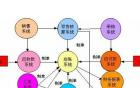# 广州天河区公司房产税如何征收

（原标题： 广州天河区公司房产税如何征收）1、收款方为个人，房屋用途为住宅、公寓、成套住宅

1）月租金800以下：例年租金 800*12=9600元

计税方法：   营业税         9600*1.5%=144元

城建税         144*7%=10.08元

教育附加       144*3%=4.32元

地方教育附加   144*2%=2.88元

房产税         9600*4%=384元

水利基金       9600*0.08%=7.68元

合计税款： 144+10.08+4.32+2.88+384+7.68=552.96元

综合税率为5.76%

2）月租金4000或4000以下：例年租金3000*12=36000元

计税方法： 每月： 营业税        3000*1.5%=45元

城建税        45*7%=3.15元

教育附加      45*3%=1.35元

地方教育附加  45*2%=0.9元

房产税        3000*4%=120元

水利基金      3000*0.08%=2.4元

年： （45+3.15+1.35+0.9+120+2.4）*12=172.8*12=2073.6元

个人所得税  月：（3000-172.8-800）*10%=202.72元

年：202.72*12=2432.64元

合计税款： 2073.6+2432.64=4506.24元

综合税率为12.52%

3）月租金4000以上：例年租金6000*12=72000元

计税方法： 每月： 营业税        6000*1.5%=90元

城建税        90*7%=6.3元

教育附加      90*3%=2.7元

地方教育附加  90*2%=1.8元

房产税        6000*4%=240元

水利基金      6000*0.08%=4.8元

年： （90+6.3+2.7+1.8+240+4.8）*12=345.6*12=4147.2元

个人所得税  月：（6000-345.6）*80%*10%=452.35元

年：452.35*12=5428.23元

合计税款：4147.2+5428.23=9575.43元

综合税率为13.30%

2、收款方为个人，房屋用途为商业、办公、商住

1）月租金4000或4000以下：例年租金 3000*12=36000元

计税方法： 每月：营业税         3000*5%=150元

城建税         150*7%=10.5元

教育附加       150*3%=4.5元

地方教育附加   150*2%=3元

房产税         3000*12%=360元

水利基金       3000*0.08%=2.4元

印花税         3000*0.1%=3元

年： （150+10.5+4.5+3+360+2.4+3）*12=533.4*12=6400.8元

个人所得税 月：（3000-533.4-800）*20%=333.32元

年：333.32*12=3999.84元

合计税款： 6400.8+3999.84=10400.64元

综合税率为28.89%

2）月租金4000以上：例年租金6000*12=72000元

计税方法： 每月：营业税        6000*5%=300元

城建税        300*7%=21元

教育附加      300*3%=9元

地方教育附加  300*2%=6元

房产税        6000*12%=720元

水利基金      6000*0.08%=4.8元

印花税        6000*0.1%=6元

年：（300+21+9+6+720+4.8+6）*12=1066.8*12=12801.6元

个人所得税 月：（6000-1066.8）*80%*20%=789.31元

年：789.31*12=9471.72元

合计税款：12801.6+9471.72=22273.32元

综合税率为30.94%

3、收款方为单位

无需缴纳个人所得税，综合税率为17.78%

另外，广州代理记账公司提示，由于房屋出租金额不一，有高有低，税务局为了核算方便，各个税务所也规定了各自的标准月租金### 天河区开公司怎么做账报税### 广州天河区代理做账报税的### 天河区新注册的公司是否需### 在天河区新成立公司做账报